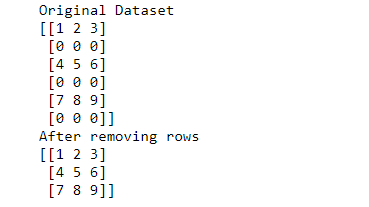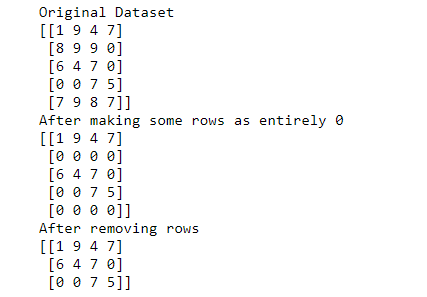# How to remove array rows that contain only 0 using NumPy?

• Last Updated : 20 Aug, 2021

Numpy library provides a function called numpy.all() that returns True when all elements of n-d array passed to the first parameter are True else it returns False. Thus, to determine the entire row containing 0’s can be removed by specifying axis=1. It will traverse each row and will check for the condition given in first parameter.

Example:

```data=[[1,2,3]
[0,0,0]
[9,8,7]]
After removing row with all zeroes:
data=[[1,2,3]
[9,8,7]]```

Example 1:

Approach Followed:

• Take a numpy n-d array.
• Remove rows that contain only zeroes using numpy.all() function.
• Print the n-d array.

## Python3

 `import` `numpy as np``# take data``data ``=` `np.array([[``1``, ``2``, ``3``], [``0``, ``0``, ``0``], [``4``, ``5``, ``6``],``                 ``[``0``, ``0``, ``0``], [``7``, ``8``, ``9``], [``0``, ``0``, ``0``]])``# print original data having rows with all zeroes``print``(``"Original Dataset"``)``print``(data)` `# remove rows having all zeroes``data ``=` `data[~np.``all``(data ``=``=` `0``, axis``=``1``)]` `# data after removing rows having all zeroes``print``(``"After removing rows"``)``print``(data)`

Output:Example 2:

Approach Followed:

• Take 20 random numbers between 0-10, using numpy.random.choice() method.
• Align them in rows and columns, using reshape() method.
• Explicitly mark some rows as completely 0.
• Remove rows having all zeroes.
• Print dataset.

## Python3

 `import` `numpy as np``# take random data` `# random.choice(x,y) will pick y elements from range (0,(x-1))``data ``=` `np.random.choice(``10``, ``20``)` `# specify the dimensions of data i.e (rows,columns)``data ``=` `data.reshape(``5``, ``4``)` `# print original data having rows with all zeroes``print``(``"Original Dataset"``)``print``(data)` `# make some rows entirely zero``data[``1``, :] ``=` `0`  `# making 2nd row entirely 0``data[``4``, :] ``=` `0`  `# making last row entirely 0` `# after making 2nd and 5th row as 0``print``(``"After making some rows as entirely 0"``)``print``(data)``data ``=` `data[~np.``all``(data ``=``=` `0``, axis``=``1``)]` `# data after removing rows having all zeroes``print``(``"After removing rows"``)``print``(data)`

Output:My Personal Notes arrow_drop_up